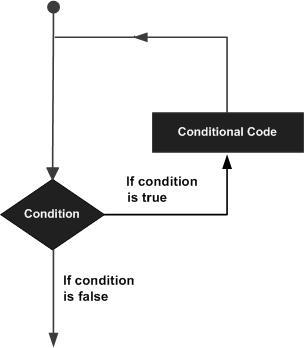Search

# For Loop Explained with Syntax and Examples of C programming

In general, statements in a program are executed sequentially. The first statement is executed first, followed by the second, and so on. But in some situations, it is required to execute a block of code over and over again. Loops are used to execute a block of statements a specified number of times until a condition is met.

### C supports the following types of loops:

• for loop

• while loop

• do-while loop

## FOR LOOP: -

A "for" Loop is used to repeat a specific block of code (statements) a known number of times.

Syntax:for (initialize counter; condition; increment counter)

{

statement(s);

}

### The flow of control in a 'for' loop is as follows:

• The initialize counter step is executed first, and only once. This step allows you to initialize any loop control variables.

• Next, the condition is evaluated. If it is true, the body of the loop is executed. If it is false, the body of the loop does not execute and the flow of control jumps to the next statement just after the 'for' loop.

• After the body of the 'for' loop executes, the flow of control jumps back up to the increment statement. This statement allows you to update any loop control variables.

• The condition is now evaluated again. If it is true, the loop executes and the process repeats itself (body of the loop, then increment step, and then again condition). After the condition becomes false, the 'for' loop terminates.

Examples:

1. To print the first 100 numbers.

#include<stdio.h>

int main()

{

int n;

for(n=1; n<=100; n++)

printf("%d\n",n);

return 0;

}

2. To print the numbers from 100 to 1.

#include<stdio.h>

int main()

{

int n;

for(n=100; n>=1; n--)

printf("%d\n",n);

return 0;

}

3. Multiplication table of any number.

#include<stdio.h>

int main()

{

int n,i;

printf("Enter a number");

scanf("%d",&n);

printf("\nMultiplictiontabe of %d",n);

for(i=1; i<=12; i++)

printf("\n%dX%d=%d",n,i,n*i);

return 0;

}

4. To print all the even numbers between 20 and 80.

# include<stdio.h>

int main()

{

int n;

printf("Even numbers between 20 and 80");

for(n=20; n<=80; n+=2)

printf("\n%d",n);

return 0;

}